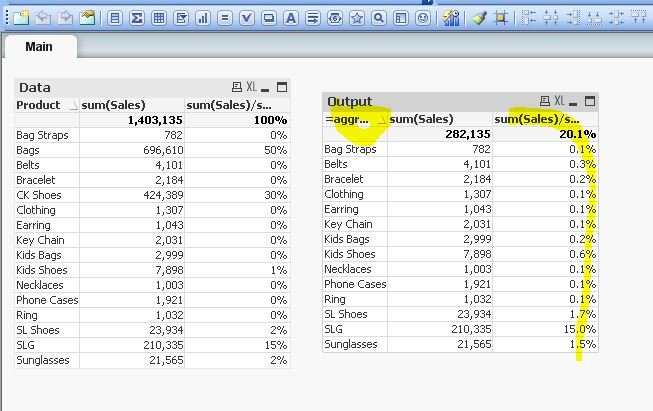# QlikView App Development

Discussion Board for collaboration related to QlikView App Development.

New Contributor III

## adding values below 25% of the total average to a chart using an if statement

I have an excel file with 400 values and I only want to display the values that are 25% or less of the total average value on a Qlik Sense chart / table.using an if statement

For example, if I have an total average value of 100 I want to display all values of 25 and less.

Tags (2)
1 Solution

Accepted Solutions
New Contributor III

## Re: adding values below 25% of the total average to a chart using an if statement

Hi All

I got the answer I needed as follows and unchecking the include null values tab

aggr(if (avg(Sales)/avg(TOTAL Sales) <0.25, Sales),Sales)

thanks for your help

10 Replies
Honored Contributor II

## Re: adding values below 25% of the total average to a chart using an if statement

Hi,

you can try to create calculated dimension like below,

for example, you have Sales and Product.

=aggr(if (sum(Sales)/sum(TOTAL Sales) <0.25, Product),Product)

(u can just apply above expression in qliksense as well and it should work) // best way try to apply this in set analysis expressionNew Contributor III

## Re: adding values below 25% of the total average to a chart using an if statement

Hi Devarasu,

thanks for your response

I tried the formula using Qlik sense and it added a column with the Product names  If I try the following

=aggr(if (sum(Sales)/sum(TOTAL Sales) <0.25, Sales),Sales)

i get a column with the Sales values. and the table is not limited to the products of 25% or below.

Any suggestions?

Valued Contributor III

## Re: adding values below 25% of the total average to a chart using an if statement

Maybe you should try by adding another parentesis:

aggr(if ((sum(Sales)/sum(TOTAL Sales)) =<0.25, Sales),Sales)

Thiago Justen Teixeira Gonçalves
WhatsApp: 24 98152-1675
Skype: justen.thiago
Honored Contributor II

## Re: adding values below 25% of the total average to a chart using an if statement

Hi,

have your tried like this?

Steps,

simply create any bar/table/pivot table chart based on your requirement

add below Calculated dimension and measure

Dimension:

=aggr(if ( (sum(Sales)/sum(TOTAL Sales)) =<0.25, Product),Product)

note: Make sure to Suppress null value in this calculated dimension.

Measure:

Sales:

Sum (Sales)

Sales Contribution %

Sum(Sales)/sum(Total Sales)

Thanks, Deva

New Contributor III

## Re: adding values below 25% of the total average to a chart using an if statement

I'm getting a new column with no values, but no errors detected in the formula and when I uncheck the include null values box all rows disappear. I changed the position of the = sign to <= but get a value that makes no sense and shows a value that is approx 11% of the sales value!!!

Any other suggestion?

Honored Contributor II

## Re: adding values below 25% of the total average to a chart using an if statement

Hi,

Can you share the mock data and expected out in excel format. i've tested my end it's working.

New Contributor III

## Re: adding values below 25% of the total average to a chart using an if statement

Hi Devarasu,

I can't attach an excel file but extract below should help

Basically what I want to show on the table is only the products where the % of average sales is 25% or below. Using your expression the column shows the "-" symbol instead of a value and shows all 400 rows regardless of the % of average sales

thanks again for your help !

 Product Sales % of sales % of average sales Multi Game Poker \$5,548.63 0.01% 5.16% Multi Game Poker \$6,427.97 0.01% 5.97% Multi Game Poker \$6,972.38 0.02% 6.48% Multi Game Poker \$7,200.70 0.02% 6.69% I love Lucy Channel Surfing \$7,465.03 0.02% 6.94% Multi Game Poker \$7,820.67 0.02% 7.27% Multi Game Poker \$7,923.34 0.02% 7.36% Multi Game Poker \$8,010.28 0.02% 7.44% Gorilla Chief II \$8,227.45 0.02% 7.64% American Roulette Deluxe \$8,353.71 0.02% 7.76% Royal Derby \$9,118.35 0.02% 8.47% Royal Derby \$9,209.20 0.02% 8.56% Multi Game Poker \$9,837.68 0.02% 9.14%
New Contributor III

## Re: adding values below 25% of the total average to a chart using an if statement

I just added an Excel file to the original post with all 400 rows

Valued Contributor

## Re: adding values below 25% of the total average to a chart using an if statement

aggr(if (sum(Sales)/sum(TOTAL Sales) <0.25, Sales),Sales)